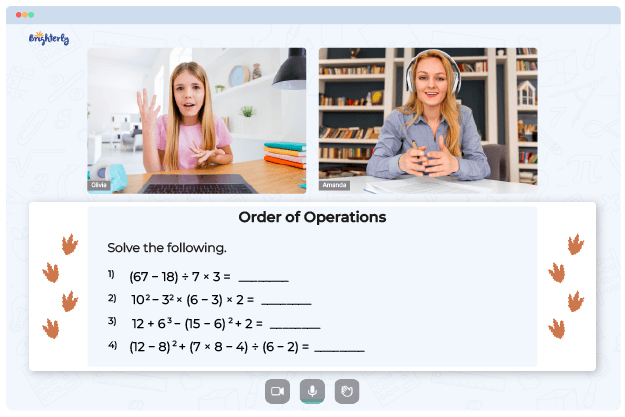# 6th Grade Order Of Operations Worksheets

The ability to solve real-world arithmetic problems depends on knowing the order of operations; therefore, all pupils must learn it. Practice with the order of operations 6th grade worksheet is a great approach to mastering the topic.

## Free order of operations worksheet 6th grade

Solving mathematical equations involves following the PEMDAS rules. When you follow the mathematical rules of operation, you’re reminded that an equation is not just an abstract assemblage of symbols but a meaningful sentence. In solving an equation, it’s crucial to perform operations in the correct sequence to retain the problem’s meaning.

Despite having the acronym “PEMDAS” to assist us in remembering the sequence of operations, it is easy to forget the order when solving an equation. You can help your kids avoid this by getting plenty of order-of-operations question practice through the order of operations worksheets with answers grade 6.

Math for Kids

Is Your Child Struggling With Math?
1:1 Online Math Tutoring## Benefits of the order of operations worksheet grade 6

Students may learn the proper method of solving arithmetic problems by working through the questions in the order of operations worksheet.

There are countless benefits of using the order of operations worksheets. These worksheets enhance the learning process by making it enjoyable. Use of these resources by students improves their critical thinking and problem-solving skills.### 6th Grade Order Of Operations Worksheets PDF

6th Grade Order Of Operations Worksheet### 6th Grade Order Of Operations Worksheets PDF

Order Of Operations 6th Grade Worksheet### 6th Grade Order Of Operations Worksheets PDF

Order Of Operations Worksheet 6th Grade### 6th Grade Order Of Operations Worksheets PDF

Order Of Operations Worksheet Grade 6

### Order of Operations Worksheets

Struggling with Order of Operations?• Does your child need extra help with understanding order of operations?
• Start studying with an online tutor.

Is your child finding it hard to grasp order of operations? An online tutor could be of assistance.